## Introduction

Millions of people edit Wikipedia pages, however, in average we find that only 5% contribute to 80% of their content. Such heterogeneous level of activity is reminiscent of the well-known and widely applicable law postulated by Pareto1, which states that 80% of the effects are induced by 20% of the causes. The example of Wikipedia users reported here highlights how heterogeneous the activity of their users are, with both, activity as well as degree following a power-law distribution. Indeed, heavy-tailed distributions following a power-law have been observed in variety of social systems ever since Pareto reported his observation of the extreme inequality of wealth distribution in Italy back in 18961. In recent years, due to ubiquitous computerization, networking and obsessive data collection, reports of heavy-tailed distributions have almost become a routine2,3,4,5,6. Following simple distributions such as those of wealth and income7, certain structural properties of social systems were also found to be heavy-tailed distributed. More specifically, distribution of the number of ties of a person (degree) has been shown to fall in this group for vast and still growing number of social networks8,12. Power-law degree distributions, called scalefree8, represent one of the three general properties of social networks (short distances and high clustering being the other two13). A power-law degree distribution is not only the least intuitive and surprising property, but also is the most well-studied and debated feature of networks since extensively found in the late 90 s8,14.

Immediately following the empirical measurements, a number of plausible models aiming at explaining the emergence of these distributions have been proposed8,9,10,11,15,16. Many models reproduce heterogeneous connectivity by amplifying small differences in connectivity – frequently stochastically emerging – using some kind of multiplicative process or “preferential attachment”8,9,10,11,15,16,17,18. Other models propose different optimization strategies leading to scale-free19,20,21. A common attribute of all these models is that fat-tailed distributions emerge out of some kind of interaction between the basic system's elements. In fact, the question is not whether there exists a mechanism that could produce scale-free networks similar to the ones observed, but which of the many mechanisms suggested are more likely to actually play a significant role in each network formation.

The data presented here suggests that there is a different underlying cause for heavy-tailed degree distributions which does not involve interactions between people. We investigate distinct social networks focusing on the relationship between users' activity and degree, specifically, the number of posts, messages, or actions of a user, i.e. activity and the number of user establishing a link with her/him, i.e. the incoming degree, or degree, for short. Both, degree k in the social network and the activity A of a user, exhibit power-law distributions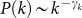and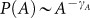, where γk and γA are the scale-free degree and activity exponents, respectively. Positively skewed distributions of human activity were recently reported22,23 and we extend this result here for a number of datasets. More importantly, in all instances we find that activity causally determine degree of the same user, suggesting that the broad distribution of one, could result from the broad distribution of the other. It is important to note that the studied actions are not likely to be driven by interaction with other people. Activity and degree, as measured here, are taken from two different networks developed by the same pool of users and so there is no reason to expect that they should depend on each other in some trivial fashion. Surprisingly, however, the number of potential followers of a user (degree distribution) appears to be entirely random except for its mean value, which is tightly controlled by the volume of activity of that user. Our observations convincingly point at the intrinsic activity of people as the driving force behind the evolution of the examined social systems and particularly the heterogeneity in user connectivity. The observed degree distribution in social systems may merely be a manifestation of the similarly wide distribution of human activity related to the system construction. These wide distributions in social collaborative networks cannot be explained by interactive model since the observed actions are not likely to be caused by actions of other people.

## Results

### Network construction

We have analyzed activity of individuals over time collaboratively working on construction of extensive electronic data sets: Wikipedia in four different languages (http://www.wikipedia.org) and a collaborative news-sharing web-site (http://www.news2.ru). These datasets represent various domains of human activity and contain records of a vast number of individual user contributions to the collaboratively generated content (see Method). For each person, we analyze two properties defined in two independent layers: activity and degree. For instance, in Wikipedia, the activity performed by users includes posting of new material and discussions about them. This is the activity layer. Simultaneously, by tracing users contributing to other users' personal or talk pages, we recover the underlying network of Wikipedia contributors' personal communication or social network. The resulting network reliably represents actual interactions of Wikipedia users24,25,26 and thus defines the social network layer. The number of incoming connections, i.e. others reaching out to the user in this network represents the degree. In principle, activity and degree as defined here are unrelated. Similarly, news2.ru posses the same two-layer structure of activity and degree (see Method).

### Analysis of activity and degree distribution

We start by analyzing the distributions of various types of activities performed by users in these systems. Very few of the most active users perform the vast majority of work so that the activity levels frequently span five orders of magnitude (Fig. 1a,b). For instance, when analyzing the activity to a given Wikipedia page, only 5% of users contribute 80% of the edits (Fig. 5 in Method). This surprising result is similar to the 80–20 rule postulated by Pareto1 to describe the unequal distribution of wealth. Indeed, a power-law faithfully characterizes the activity distributions in Fig. 1. The exponent of the activity distribution for Spanish language Wikipedia is γA = 1.752 ± 0.005 (Fig. 1a), while the activity distribution for voting in stories in News2.ru is γA = 1.88 ± 0.04 (Fig. 1b, detailed fitting procedure in Method32,33)

The activity distributions in Fig. 1a represent the number of users as a function of the number of Wikipedia edits in four languages. Interestingly, different populations performing similar activity in separate instances of similarly-built social systems exhibit identical activity distributions. Figure 1b shows several different activities performed by the same population of users at the social news aggregator news2.ru. These activities differ in their complexity. We consider submission of posts to be the most difficult and time consuming of the five activity types because it typically requires the user to locate the content on-line, evaluate its quality and publish at the news2.ru web site by filling a form with multiple fields. Considering the task complexity, writing comments is arguably easier task than posting. There are on average nearly three comments per every published post. These two content-generating tasks are followed by ranking of posts and comments. The differences in the underlying complexity of the task seem to explain the difference in the range and slope of the observed distributions plotted in Fig. 1b.

We further observe the social networks emerging in each of these systems. These networks serve different functions. In Wikipedia they arise due to the direct interaction required to coordinate common tasks. In particular, we derive social networks from the record of edits of personal user pages by other users - a common way of personal communication in Wikipedia (the web site rules forbid activity-related confidential communication between its editors). In news2.ru the social network emerges through declaration of personal attitudes - a user may indicate that he/she likes, dislikes or is neutral to any other user. Another social network arises from a set of explicit (directed) declarations of friendship between news2.ru users. Figure 1 c and d present the degree distributions in these networks. Broad distributions are measured and present in each system, suggesting a scale-free behavior in their degree distribution. The exponent of the degree distribution for Spanish Wikipedia is γk = 1.92 ± 0.01 (Fig. 1c) and for the degree distribution in News2.ru is γk = 2.11 ± 0.08 (Fig. 1d).

### Dependence between activity and degree

The present data suggest a simple explanation of the origin of degree distributions. We first observe that the number of incoming links aggregated by a person in all these social networks is highly correlated to the individual's activity. The correlation between the degree and the activity measurements is presented in Table I. It is measured here as the correlation of the log-values to capture the gross relationship of these two variables across different orders of magnitude. More importantly, the dependence analysis below suggests that the broad distribution of activity is the driving force of scale-free degree as will be discussed next.

It is important to emphasize that in order to avoid direct and rather obvious correlation between different aspects of activity of the same person, we test the correlation of individual's activity to her degree determined by actions of his/her followers rather than his/her own. It is possible that these actions are driven by reciprocity, i.e., a person is simultaneously active in the community and in constructing her social network inspiring others to link back to her.

To determine the precise nature of the (k, A) relationship, we analyze the joint distribution of degree and activity, p(k, A) (Fig. 2a). We find that the mean degree μk for a given level of activity follows a smooth monotonic function of A (Fig. 2b), whereas the opposite is not true, i.e., the mean activity μA does not seem to be tightly determined by degree (Fig. 2c). A similarly tight relationship exists for the standard deviation of the degree distribution σk for specific values of the activity (Fig. 2d), but, again, the reverse is not true (Fig. 2e). The conditional mean and standard deviation of degree (conditioned on activity) show a tight relationship with approximately unit slope σk ≈ μk (Fig. 2f). However, the σA, μA values conditioned on degree are more variable (Fig. 2g). Based on these observations we hypothesize that the conditional degree distribution p(k|A) may be scale invariant with scale μk entirely determined by activity: μk = f(A). Here, this functional dependence of scale can be estimated as the mean activity for a given A: μk = f(A) ≈ mean(k|A). Indeed, we observe that the conditional degree distribution appears to follow a geometric distribution for all μk: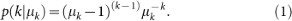This theoretical distribution provides a remarkably accurate fit to the first two sample moments of degree for a given level of activity as shown in Fig. 3. We plot the standard deviation σk versus mean degree μk for given activity for four Wikipedia databases. The curves follow a smooth, monotonically increasing functional form which is almost identical for all datasets (as one would expect for activity conditioning degree). When the analysis is repeated for activity conditioned on degree the variables do not appear to follow a tight relationship.

The tight relationship between σk versus μk conditioned on activity follows asymptotically a straight line with unit slope, which follows exactly the geometric distribution Eq. (1). In Fig. 3, we compare the data to the analytic relationship between mean and standard deviation for geometric distribution Eq. (1):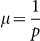and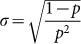, where p is the parameter of geometric distribution. The data fit this theoretical curve surprisingly well for the four displayed languages of Wikipedia (r2 = 0.8889 in average).

### Dependence hypotheses

The previous findings can be understood with the following hypothesis H1: Ak, activity deterministically affects the mean degree, but degree is otherwise random (Fig. 4a). Note that for positive discrete variables – like the degree – with a given mean, the highest entropy or least informative and most random distribution is achieved by the geometric distribution as we find above27. The geometric distribution is analogous to exponential distribution in statistical mechanics, which maximizes entropy for continuum variables with fix mean. We also tested the inverse hypothesis H2: kA, degree deterministically affects mean activity, μA = g(A) ≈ mean(A|k) and activity is otherwise random.

The goodness-of-fit of these two analytic models to histograms of H1: activity → degree or H2: degree → activity was measured with the χ-square statistics averaged over activity or degree respectively. The likelihood that the observed distributions match H1 or H2 was assessed using surrogate data generated with Monte-Carlo sampling to estimate the chance occurrence of these averaged χ-square values. The results for the Spanish language Wikipedia data indicate that we cannot dismiss the correctness of H1 (Fig. 4b) with a confidence of higher than 95% (p = 0.23) but that H2 can be soundly dismissed (the chance of the corresponding χ-square value occurring at random is p < 10−5). The same is true for all other datasets (see Table I). In all datasets the likelihood of H1 is several orders of magnitudes larger than H2 and thus we accept model H1, which states that activity determines degree.

Given the explicit model of a geometric distribution for P(k|A) of hypothesis H1 and the observed power-law distribution for activity,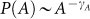, one can explicitly derive the expected degree distribution. The conditional degree distribution closely matches a geometric distribution (Fig. 3). For large mean values, say μk > 10, it can be very well approximated by its continuous equivalent, the exponential distribution i.e.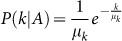. Therefore: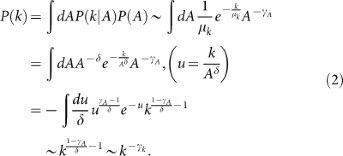Thus the exponent is predicted to be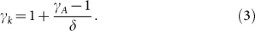where δ defines μk ~ Aδ for large A as shown in Figure 2b. The observed exponents γk closely follow these predicted exponents for all datasets (Table I).

## Discussion

The causal inference argument provided here is borrowed from ideas recently developed in causal inference28,29,30. There, a deterministic functional dependence of cause on mean effect is hypothesized and deviations from this mean effect are assumed to have fixed standard deviation but to be otherwise random. With two variables for which one wishes to establish causal direction, the model is evaluated in both directions and the more likely one is postulated to indicate the correct causal dependence, as we have done here. This approach has been demonstrated to give the correct causal dependence for a large number of known causal relationships31 and theoretical results indicate that there is only an exceedingly small class of functional relationships and distributions for which this procedure would give the incorrect answer. Such an identifiability proof does not yet exist for the present case where the standard deviation is not constant. Nevertheless, our explicit model of a deterministic effect of human activity on the success of establishing social links is the simplest possible explanation for the data available to us. For a different dataset a different probabilistic model may be better suited.

The individual activity of people deterministically affects the mean success at establishing links in a social network and the specific degree of a given user is otherwise random following a maximum entropy attachment (MEA) model. The MEA model is exemplified in Fig. 4a and consists of the following steps: Introduce a node i with q links, where q is drawn from a probability given by the activity of the node. The activity has an intrinsic power-law distribution. Then, link the q links at random following maximum entropy principle with the concomitant geometric distribution P(kk). This mechanism contrasts with the preferential attachment mechanism8,15,16,17,18 where each link attaches to a node with a probability proportional to the number of links of that node. A possible mechanism by which a geometric distribution could arise is based on the notion of “success”. In this model, the activity of users aims to achieve a specific outcome (a Wikipedia project) and each new incoming link can aid in achieving this desired outcome; once the goal is achieved the user stops collecting links. The probability of the desired event in this model is q = 1/μk ~ A−δ. Hence, those users working so very hard may have an exceedingly unlikely event they are aiming for. But eventually, they too will succeed and will turn their attention away from the on-line social network.

The present data indicates that degree distribution is maximally random except for what can be determined solely from the volume of a user's activity. Does this mean that the precise content of a user's actions (the meaning and quality of the edits in Wikipedia, messages, etc) is immaterial in determining his/her success in establishing relationships? One can only hope that small deviations from this maximum entropy attachment model will become more pronounced with increasing data-set sizes, which can then point us to the benefits of well thought out and carefully executed actions, specially in specialized large-scale collaborative projects like Wikipedia.

Whether the dynamics of preferential attachment is consistent with the maximum entropy distribution of degree remains to be established. What is certain is that distributions of levels of activities in all tested populations are heavily heavy-tailed indicating highly varying level of involvement of users in collaborative efforts. We showed here that this fact alone is sufficient to produce the heavy-tailed distribution of degree observed throughout social networks. Therefore, previous interactive models may not be necessary. The present result shifts the burden of proof to explaining the origin to the incredible diversity in human effort observed here spanning five orders of magnitude.

## Methods

### Datasets

The number of actions contained in the datasets range from hundreds of thousands to hundreds of millions of user actions. From the editing on Wikipedia, to the votes, to commentaries on News2.ru, these actions represents different and natural underlying dynamics of social networks, since they range from collaborative interaction (Wikipedia) to discussions about different interesting of human behavior (New2.ru), which are intrinsic properties of the social nature of the web.

We have collected details about user activity in the Wikipedia project and reconstructed the underlying social network. In addition to the widely used term and category pages, Wikipedia provides special pages associated with specific contributing authors and discussion (talk) pages maintained alongside each of these pages. These user pages are widely used by Wikipedia contributors for coordination behind the scenes of the project. In fact, interaction via user and discussion pages dominates all other communication methods. However, communication via personal user pages (and the corresponding discussion pages) differs from the topic-associated talk pages in that it is explicit person-to-person communication rather than general topic specific, usually impersonal communication. By tracing users contributing to other user's personal or talk pages, we recover the underlying network of Wikipedia contributor's personal communication. Not surprisingly, as presented in the next section, the obtained social networks show a scale-free degree distribution, typically observed in a variety of social networks analyzed so far.

The other data set is a de-identified record of activities of social news aggregator news2.ru. The record contains all actions performed by the community members over more than three years of collaborative selection and discussion of news-related content. These, user-related actions include such events as submission of news article, comments as well as preference-revealing actions such as voting for articles (“dig” or “bury”, using digg.com language) and other users' comments. In addition to the trace of user activity, the data contains explicit social network layer. Each user may publicly declare his/her (positive, neutral or negative) attitude to any other user. Considering the personal flavor of the rather emotional way people interact through commentary threads, this list of attitudes when aggregated can be perceived as social network. In addition, users maintain list of friends, usually including users most favorable on them. These networks are directional, which allows to focus on the incoming links, since they can not be controlled by the target individual, but by his/her friends.

Each of these systems represents different approaches to collaborative content creation. The Wikipedia editors interact to create the same content collaboratively so that the content contributed by one user can be complemented, altered or completely removed by others. The news2.ru represents a mixed case in which the content is contributed individually, but collaboratively ranked. Given these fundamental differences in user activity and network dynamics, the similarities between these systems reported below are particularly revealing.

### Method of power-law fitting

To get the exponents γk and γA of power-law distribution, we present a rigorous statistical test based on maximum likelihood methods32. Take the degree distribution as an example. We fit degree distribution assuming a power law within a given interval. For this, we use a generalized power-law form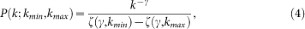where kmin and kmax are the boundaries of the fitting interval and the Hurwitz ζ function is given by ζ(γ, α) = Σi(γ + α)−γ.

We use the maximum likelihood method, following the rigorous analysis of Clauset et al.32. The fit was done in an interval where the lower boundary was kmin. For each kmin value we fix the upper boundary to kmax = K, where K is the maximal degree. We calculate the slopes in successive intervals by continuously increasing kmin and varying the value of w. In this way, we sample a large number of possible intervals. For each one of them, we calculate the maximum likelihood estimator through the numerical solution of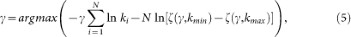where ki are all the degrees that fall within the fitting interval and N is the total number of nodes with degrees in this interval. The optimum interval was determined through the Kolmogorov-Smirnov (KS) test.

For the goodness-of-fit test, we use the Monte Carlo method described in32. For each possible fitting interval, we calculate the Kolmogorov-Smirnov statistics D for the obtained cumulative distribution function. Then we choose the interval with the minimal D as the best fitting interval and take the γ in this interval as the final result. As to the standard error estimation, we adopt the method in32. The standard error on γ, which is derived from the width of the likelihood maximum, is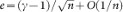, where n is the number of data.

Although the fitting method mentioned above is rigorous, it is suitable for fitting probability density distributions. When we fit the data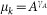, we use another fitting method33. The procedure for determining fitting interval is similar. In each fitting intervals, the fittings were done using ordinary least squares methods. The goodness of fitting was estimated through the coefficient of determination, r2, where 0 ≤ r2 ≤ 1. The value of r2 is used as a measure of how reliably the fitted line describes the observed points and is often described as the ratio of variation that can be explained by the fitted curve over the total variation. We assume that any value above r2 ≥ 0.85 represents an accepted fitting. The final result is the average of the accepted exponent.